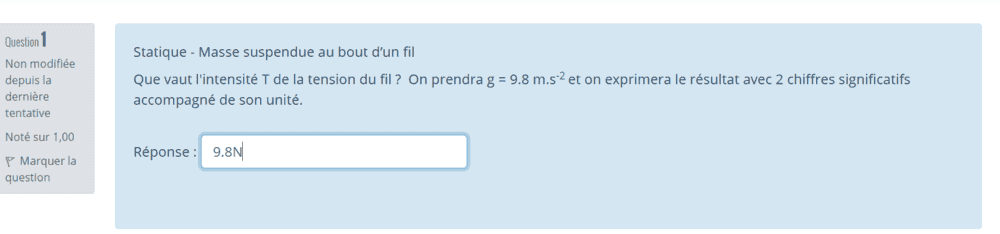# Point mechanics -- Tension in a rope from a hanging mass

Homework Statement:
Rope tension
Relevant Equations:
t = ma
Hi,
I'm being asked to determine the tension of a rope only knowing that g = 9.8 m.s-2. I understand that in order to calculate tension, I would need to multiply mass with acceleration. But i dont understand how i would in this case. This is the question for reference. Thanks for your help.
And 9.8N isnt correct so im confused..Last edited by a moderator:

Lnewqban
Gold Member
What is the value of the mass in Kg?

kuruman
Homework Helper
Gold Member
Is this all that is given? The tension depends on the mass and if the mass is not given, then you cannot find a numerical answer for the tension.

What is the value of the mass in Kg?
This why I don't understand the question because the mass is not given.

Is this all that is given? The tension depends on the mass and if the mass is not given, then you cannot find a numerical answer for the tension.
I was thinking if there is somehow i could write the tension algebraically somehow I don't know?

kuruman
Homework Helper
Gold Member
I was thinking if there is somehow i could write the tension algebraically somehow I don't know?

If the mass is not given, then the question cannot be answered. If the mass is not accelerating, the tension is equal to the weight ##mg## which is the product multiplied by the acceleration of gravity. I doubt that an algebraic answer is expected. You are told to write the answer to two significant figures (2 chiffres significants) which means numbers, n'est-ce pas?

•Lnewqban
If the mass is not given, then the question cannot be answered. If the mass is not accelerating, the tension is equal to the weight ##mg## which is the product multiplied by the acceleration of gravity. I doubt that an algebraic answer is expected. You are told to write the answer to two significant figures (2 chiffres significants) which means numbers, n'est-ce pas?
Yess with 2 significant figures ( I didn't know that's how it was called btw so thanks a lot). So this might be a mistake from the prof right? Cuz I was thinking something like m.9.8 but it's not correct.

berkeman
Mentor
Was there any text above this Question #1? Something like, "in the following questions, use m = 2kg"...

Steve4Physics
Homework Helper
Gold Member
EDIT. Just realised that you have to enter your answer into a computer. So my suggestion (below) is inapplicable. Sorry.

If you are sure that you have the full, correct question, and that no value of mass is given, you could write something like this (but in French I presume!):

"Since no value of mass is given, for the purpose of showing the method I will take the mass to be 17kg."

And then you can complete the question.

You should then get full credit.

kuruman
Homework Helper
Gold Member
You should then get full credit.
Not if the algorithm that scores the answer expects a specific numerical value. Better ask the person who assigned the problem for clarification.

On edit:
EDIT. Just realised that you have to enter your answer into a computer. So my suggestion (below) is inapplicable. Sorry.
And I just realized that you have rescinded your suggestion which makes my comment (above) unnecessary. Sorry.

Last edited:
Ok I see. Thanks so much for the help!

•Delta2 and kuruman